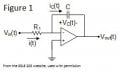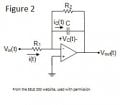# Ideal vs practical op amp help

#### joepdon

Joined Jan 19, 2016
10
The lab I am working on is about ideal and practical op amps. We started off wiring up an ideal op amp for just one step and measured the output voltage before setting up the practical op amp. In the lab report I am suppose to discuss what I observed when doing this and explain why the ideal op amp integrator is not a practical circuit. It then says to note the amount of feedback that exists in the ideal integrator. Then it asks for the importance of adding a feedback resistor in parallel with the capacitor creating a practical ideal integrator.

I know the difference between the ideal and practical, but I guess I just don't understand what I am looking at. The voltage output for the ideal was 11.5V and with adding the feedback resistor which is double the input resistor I saw 13.1mV. Can someone help me realize what went on. Keep in mind this is my second circuits class and I haven't taken a circuits and differential equation class for about a year so things might need to be broke down into baby steps.
Thanks.#### WBahn

Joined Mar 31, 2012
28,172
Where did you find an ideal op amp to wire up in a lab course?

•ErnieM

#### joepdon

Joined Jan 19, 2016
10
I guess we do not have one we just use the lm741 and wire it up as seen in figure 1 and call it ideal? Cause there is no real life ideal op amp right?

#### RBR1317

Joined Nov 13, 2010
706
It is likely that the ideal/non-ideal label is not referring to the op-amp itself but rather to the circuit design which assumes what the op-amp that is used will be. So using a 741 in a circuit that was meant for an ideal op-amp can lead to some really bad performance results.

#### joepdon

Joined Jan 19, 2016
10
I think that is what my professor is doing, which makes sense in showing that solving it with the assumptions of an ideal op amp on paper doesn't work in real life.

#### WBahn

Joined Mar 31, 2012
28,172
Under what conditions did you measure the output? It's an integrator, so you expect it's output to be a function of the history of its input.

#### joepdon

Joined Jan 19, 2016
10
I measured the output with an oscilliscope connected to ground and the output. The op amp is biased at +/-12V, C=1nF, R1=100Kohms, R2=200kohms. Or are you asking for the equation?:
Vout(t)=-(1/(RC)) integral[Vin(t)dt - Vc(0)] from 0 to t (this is for the ideal op amp)

#### thumb2

Joined Oct 4, 2015
122
joepdon said:
The voltage output for the ideal was 11.5V and with adding the feedback resistor which is double the input resistor I saw 13.1mV. Can someone help me realize what went on
Adding a feedback resistor the circuit turns into a inverting amplifier, so the output falls down to GND (0 V).

Note that the second circuit is also a low-pass active filter.•joepdon

#### hp1729

Joined Nov 23, 2015
2,304
The lab I am working on is about ideal and practical op amps. We started off wiring up an ideal op amp for just one step and measured the output voltage before setting up the practical op amp. In the lab report I am suppose to discuss what I observed when doing this and explain why the ideal op amp integrator is not a practical circuit. It then says to note the amount of feedback that exists in the ideal integrator. Then it asks for the importance of adding a feedback resistor in parallel with the capacitor creating a practical ideal integrator.

I know the difference between the ideal and practical, but I guess I just don't understand what I am looking at. The voltage output for the ideal was 11.5V and with adding the feedback resistor which is double the input resistor I saw 13.1mV. Can someone help me realize what went on. Keep in mind this is my second circuits class and I haven't taken a circuits and differential equation class for about a year so things might need to be broke down into baby steps.
Thanks.
View attachment 99553 View attachment 99554
By Ideal versus Practical I take it you refer to one with just the cap as feedback versus one with a resistor?
Were the voltages you refer to DC or AC, If AC what frequency?

#### hp1729

Joined Nov 23, 2015
2,304
It is likely that the ideal/non-ideal label is not referring to the op-amp itself but rather to the circuit design which assumes what the op-amp that is used will be. So using a 741 in a circuit that was meant for an ideal op-amp can lead to some really bad performance results.
<>Dang. Will we ever get away from that LM741 in schools? </> It is still a good example of a bad example in op amps. The "Acme product" everybody wants to compare their product to for "lower offset ... less noise ... etc.
.

#### joepdon

Joined Jan 19, 2016
10
By Ideal versus Practical I take it you refer to one with just the cap as feedback versus one with a resistor?
Were the voltages you refer to DC or AC, If AC what frequency?
In this part of the lab we measured them in DC.

#### WBahn

Joined Mar 31, 2012
28,172
In this part of the lab we measured them in DC.
So what was the DC input voltage? 0V? 10 V? What?

#### dannyf

Joined Sep 13, 2015
2,197
Can someone help me realize what went o
The ideal integrator is likely oscillating. The integration capacitor shorts the opamp to ground and most opamps, especially a 741, would not be able in that case.

The ideal of paralleling the capacitor with a large resistor is to better define the upper end of the gain. However, in this case your choice of that resistor has too low of a value. Use a large value resistor instead.

#### dannyf

Joined Sep 13, 2015
2,197
BTW, when the circuit is working, its output will change linearly with time. Either increase or decrease depending on the flow of current.

#### joepdon

Joined Jan 19, 2016
10

#### dannyf

Joined Sep 13, 2015
2,197
I would venture a guess that the input voltage is quite low, in the 13mv range.#### hp1729

Joined Nov 23, 2015
2,304
In this part of the lab we measured them in DC.
The capacitor is an open circuit at DC. Maximum gain in the op amp and max voltage out. This the difference with the resistor across the cap.